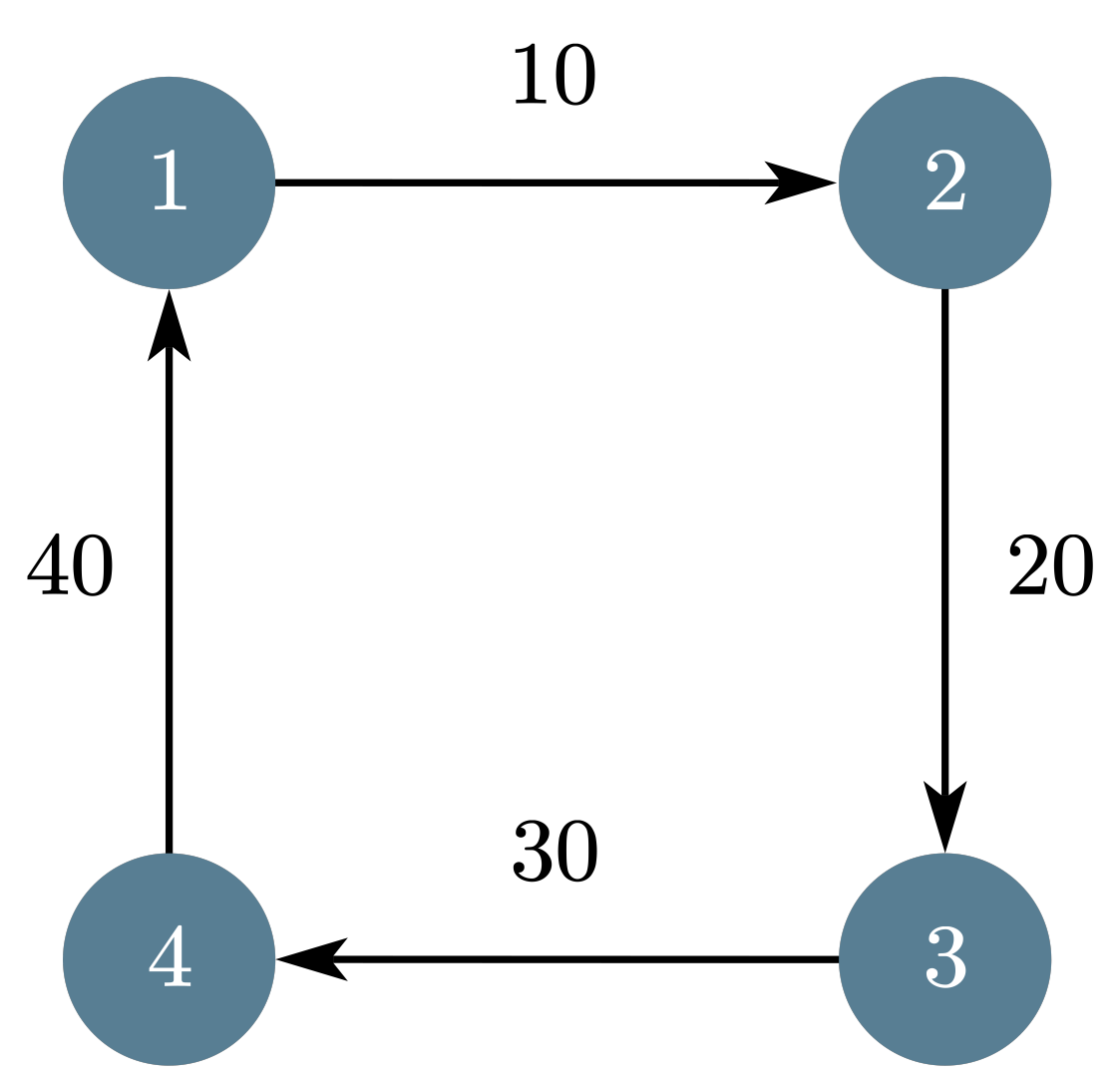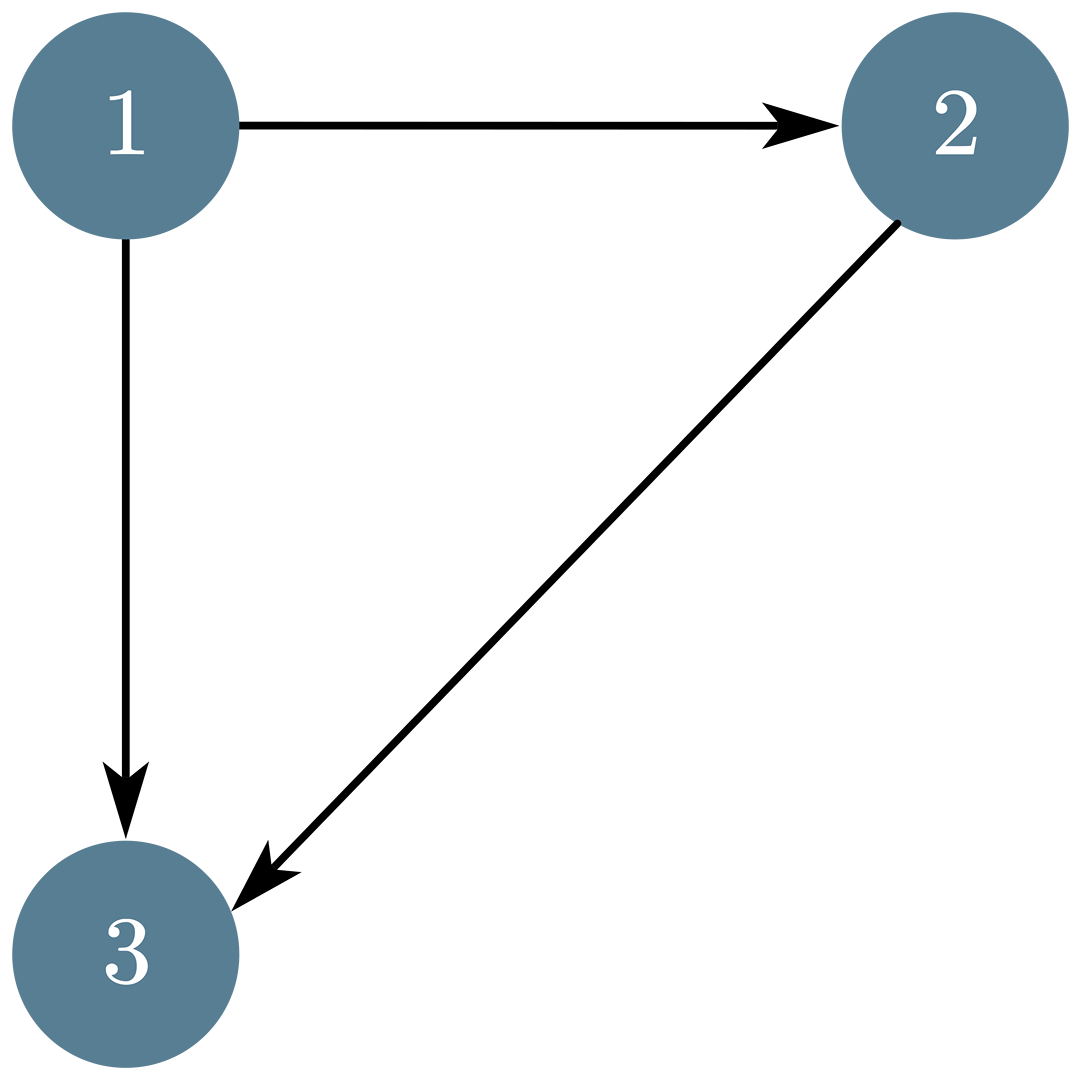# 1：题意简述

$N$ 个奶牛，奶牛 $i (1 \le N)$ 想访问奶牛 $a_i (a_i \ne i)$ 。如果 $a_i$ 已经离开去访问别的奶牛了，则 $i$ 不能成功访问 $a_i$，否则，这次成功访问可以增加 $v_i$ 次哞叫。 现在让你找出可能的最大哞叫次数

# 2：分析• 最小（大）生成树的算法只能用于无向图中，而我们当前的图是有向图，所以我们可以直接把最小（大）生成树算法用在这道题里吗

（这部分如果理解了可以直接看代码)，代码就是个标准的 kruskal# 3：代码

/*Date: 22 - 03-26 15 28
PROBLEM_NUM: USACO MAR Problem 1. Visits*/
#include <bits/stdc++.h>
using namespace std;
const int MAXN = 2e5 + 10;
#define ll long long
struct E
{
int from, to, val;
} e[MAXN];
int n;
int fa[MAXN];
int find_fa(int cur)
{
if (cur == fa[cur])
return cur;
return fa[cur] = find_fa(fa[cur]);
}
void merge(int a, int b)
{
int af = find_fa(a), bf = find_fa(b);
fa[af] = bf;
}
//并查集操作

ll ans;

int main()
{
scanf("%d", &n);
iota(fa + 1, fa + 1 + n, 1);//最开始 fa[i] = i
for (int i = 1; i <= n; i++)
{
scanf("%d%d", &e[i].to, &e[i].val);
e[i].from = i;
}
sort(e + 1, e + 1 + n, [](E a, E b)
{ return a.val > b.val; });//权值大的放前面
int used_edge = 0;
for (int i = 1; i <= n; i++)//kruskal
{
if (find_fa(e[i].from) != find_fa(e[i].to))
{
used_edge++;
ans += e[i].val;
merge(e[i].from, e[i].to);
if (used_edge == n - 1)
{
break;
}
}
}
printf("%lld\n", ans);
system("pause");
}